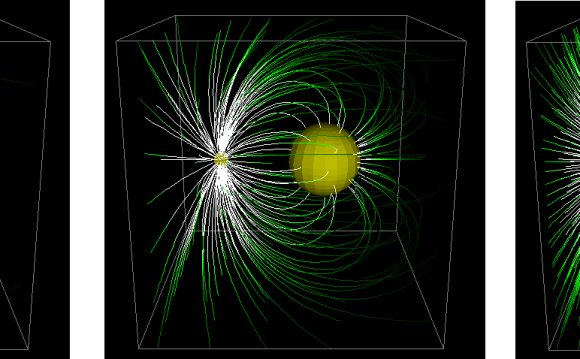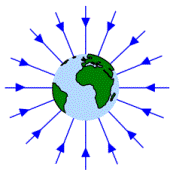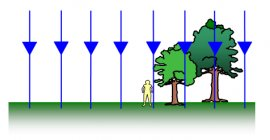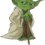# Gravitational field Lines

November 7, 2014This episode introduces the notion of a "field of force" and how we can diagrammatically represent such a field using field lines. The actual strength of the field at a point in space is defined by the field strength.

Summary

• Discussion: Fields and field lines (10 minutes)
• Discussion: Field strength, g (10 minutes)
• Worked examples: Field strength (20 minutes)
• Spreadsheet exercise: Analysing data from the Apollo 11 mission (20 minutes)

Discussion: Fields and field lines
When you pick up an object such as a pen, there is direct contact between you and the pen. This direct contact exerts a force on the pen, causing it to move in the way that it does. However, the pen also has a weight due to its presence in the Earth’s gravitational field. How is this force exerted, even when there is no direct contact between the Earth and the pen? A force is exerted on the pen from the Earth because the pen is in the Earth’s gravitational field. We can define the field due to a body as the region of space surrounding it where other bodies will feel a force due to it.

What extent do gravitational fields have? (The gravitational force is infinite in range, although it becomes very weak at large distances as it is an inverse square law. The gravitational field due to a body is thus also infinite.)

We cannot see or touch this field, but we can try to model it using field lines or lines of force. In a field line diagram, the direction of the field line at a point gives the direction of the force of attraction that would be felt by a small mass placed there. The relative density of field lines on the diagram is an indication of the strength of the field. (Compare this with the more familiar magnetic field patterns.)

Thus for a spherical mass, like the Earth, we would have the following diagram:

The field lines are directed radially inwards, because at any point in the Earth’s field, a body will feel a force directed toward the centre of the Earth. The field lines become more spread out as the distance from the Earth increases, indicating the diminishing strength of the field. Note that the field is really 3-dimensional, but of course on paper, we can only take a 2-dimensional slice of it. This is a radial or spherical field.Close to the surface of the Earth, the field lines look like:

They are directed downwards (the direction in which a body near the Earth’s surface would feel a gravitational force), and they are parallel and equidistant indicating that the field is constant, or uniform.

A couple of important points to note:

1. Field lines do not start or stop in empty space (even though on diagrams they have to stop somewhere!). They end on a mass and extend back all the way to infinity.
2. Field lines never cross. (If they did, then an object placed at the point where they crossed would feel forces in more than one direction. These forces could be resolved into one direction – the true direction of the field line there.)

(The concepts of field and field lines will be used again when we look at electric fields and magnetic fields).

Discussion: Gravitational field strength, g
As you’ve already seen, you pull on the Earth with exactly the same force as it pulls on you. However, your gravitational field is intrinsically much, much weaker than that of the Earth. To try to get a handle on the intrinsic strength of the gravitational field of a body, we need to define field strength at a point in the field.

We define field strength at a point in a body’s field as the gravitational force exerted on an object placed at that point, per kg of the object’s mass. In other words, it is just the number on newtons of attractive force acting per kg of the object’s mass. Since the attractive force is simply what we call weight, we can write this as:

g = W/m

where W = weight in newtons. Thus g has units N/kg.

We can use this definition to get an equation for using Newton’s law of universal gravitation. The attractive force of a mass M (causing the field) on a mass m a distance r away is simply GMm/r2. Thus the attractive force per kg of mass of the object (mass m) is (GMm/r2)/m.

Thus, g = GM/r2

This gives an expression for the field strength at a point distance r from a (point or spherical) mass M.

A couple of points to note:

1. The gravitational field strength at a point in a field is independent of the mass placed there – it is a property of the field. Thus, two objects of different mass placed at the same point in the field will experience the same field strength, but will feel different gravitational forces.
2. In some texts a minus sign is present in this equation, so it reads g = -GM/r2; this is from the more correct, but off syllabus, vector equation for g. It is better to calculate the magnitude of the field strength using g = GM/r2 and then the direction is given by the fact that gravity is always attractive (i.e. the field always acts towards the gravitating body).

Worked examples: Field strength
Calculations involving field strength.

Here, students can try analysing data from the Apollo 11 mission.

Source: tap.iop.org
##### INTERESTING VIDEOClass 11-Physics-Gravitation-On line IIT-JEE Video Lectures3-gravitational field linesGravitational Field

##### INTERESTING FACTS##### What is a gravitational field?

A gravitational field is a vector velocity field. Matter creates a velocity field v = (GM/r)^.5, the Gravitational potential energy is V = -mGM/r = -mv^2. The total Energy is